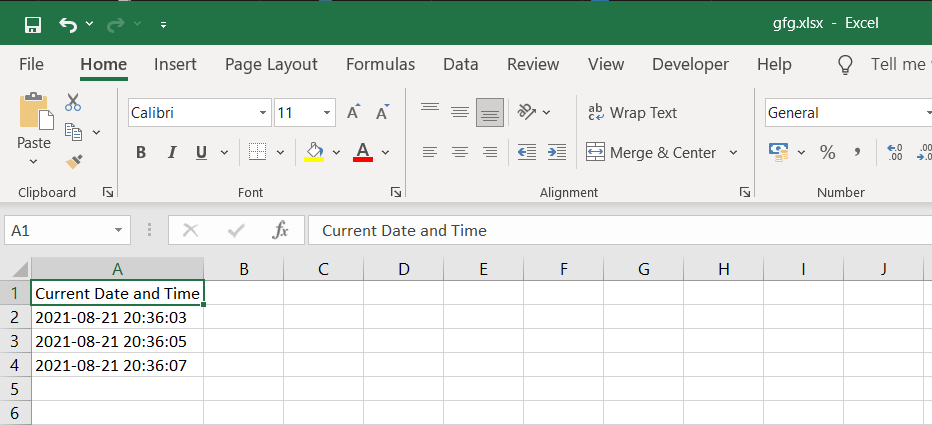# How to add timestamp to excel file in Python

• Last Updated : 14 Sep, 2021

In this article, we will discuss how to add a timestamp to an excel file using Python.

## Modules required

• datetime: This module helps us to work with dates and times in Python.
`pip install datetime`
• openpyxl: It is a Python library used for reading and writing Excel files.
`pip install openpyxl`
• time: This module provides various time-related functions.

## Stepwise Implementation

Step 1: Create the workbook object and select the active sheet:

Attention geek! Strengthen your foundations with the Python Programming Foundation Course and learn the basics.

To begin with, your interview preparations Enhance your Data Structures concepts with the Python DS Course. And to begin with your Machine Learning Journey, join the Machine Learning - Basic Level Course

```wb = Workbook()
ws = wb.active```

Step 2 (Optional): Write the heading in cell A1.

```# Here column=1 represents column A and row=1 represents first row.
ws.cell(row=1, column=1).value = "Current Date and Time"```

Step 3: Use the following command to get the current DateTime from the system.

`time = datetime.datetime.now().strftime('%Y-%m-%d %H:%M:%S')`

The strftime() function is used to convert date and time objects to their string representation. It takes one or more inputs of formatted code and returns the string representation.

Step 4: Write the DateTime in cell A2.

`ws.cell(row=2, column=1).value = time`

Step 5: Initiate a sleep of 2 seconds using time.sleep(2)

Step 6: Similarly, get the current datetime again and write it in cell A3. Here you will notice a difference of 2 seconds from the previous DateTime since there was a sleep of 2 seconds in between.

Step 7: Finally, Save the excel workbook with a file name and close the workbook

```wb.save('gfg.xlsx')
wb.close()```

Below is the full implementation:

## Python3

 `# Import the required modules``import` `datetime``from` `openpyxl ``import` `Workbook``import` `time`` ` ` ` `# Main Function``if` `__name__ ``=``=` `'__main__'``:``   ` `    ``# Create a worbook object``    ``wb ``=` `Workbook()`` ` `    ``# Select the active sheet``    ``ws ``=` `wb.active`` ` `    ``# Heading of Cell A1``    ``ws.cell(row``=``1``, column``=``1``).value ``=` `"Current Date and Time"`` ` `    ``# Cell A2 containing the Current Date and Time``    ``ws.cell(row``=``2``, column``=``1``).value ``=` `datetime.datetime.now().strftime(``'%Y-%m-%d %H:%M:%S'``)`` ` `    ``# Sleep of 2 seconds``    ``time.sleep(``2``)`` ` `    ``# Cell A3 containing the Current Date and Time``    ``ws.cell(row``=``3``, column``=``1``).value ``=` `datetime.datetime.now().strftime(``'%Y-%m-%d %H:%M:%S'``)``    ``time.sleep(``2``)`` ` `    ``# Cell A4 containing the Current Date and Time``    ``ws.cell(row``=``4``, column``=``1``).value ``=` `datetime.datetime.now().strftime(``'%Y-%m-%d %H:%M:%S'``)`` ` `    ``# Save the workbook with a``    ``# filename and close the object``    ``wb.save(``'gfg.xlsx'``)``    ``wb.close()`

Output:My Personal Notes arrow_drop_up